# Teach you how to extract tables in PDF with Python

2022-01-31 23:41:02

「 This is my participation 11 The fourth of the yuegengwen challenge 18 God , Check out the activity details ：2021 One last more challenge 」.

### Preface

`pdfplumber` It's an open source `python` Tool library , It can easily get `PDF` Text content 、 title 、 form 、 Size and other information , Today I'll show you how to use it to extract `PDF` The table in .

### install

First install... With the following command `pdfplumber` modular .

``````pip install pdfplumber
Copy code ``````

Or use watercress image source to install .

``````pip install -i https://pypi.douban.com/simple pdfplumber
Copy code ``````

### Case study

Here is a copy of 2020 List of winners of entries in China University Student Computer Design Competition , File for `PDF` Format , Each page contains a table , The table contains the award information for each team , common 158 page . The first two pages of the form are as follows .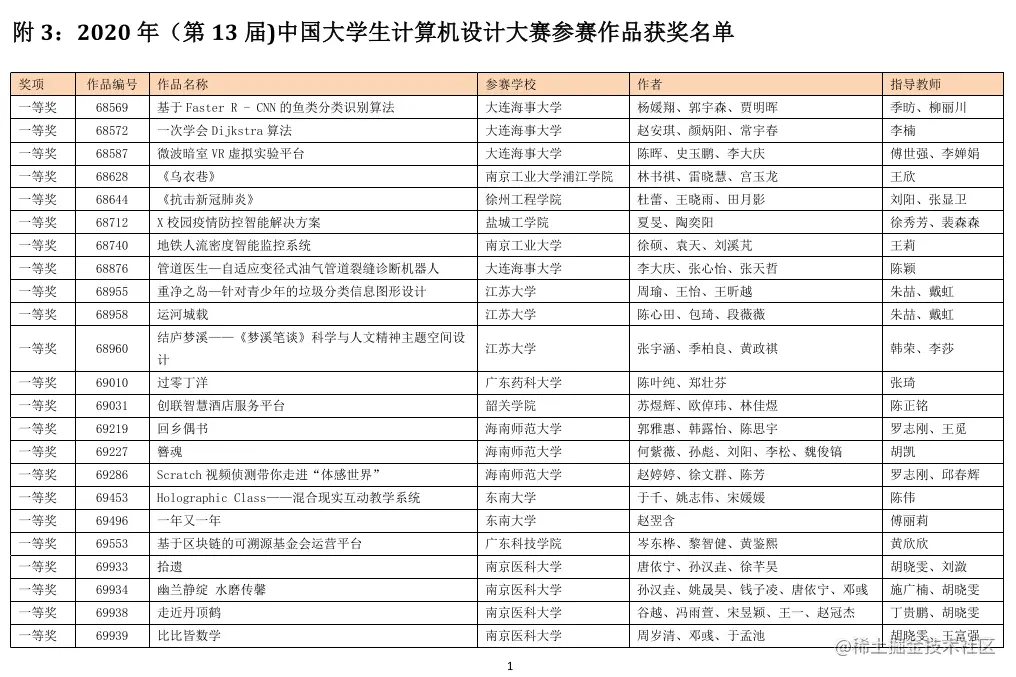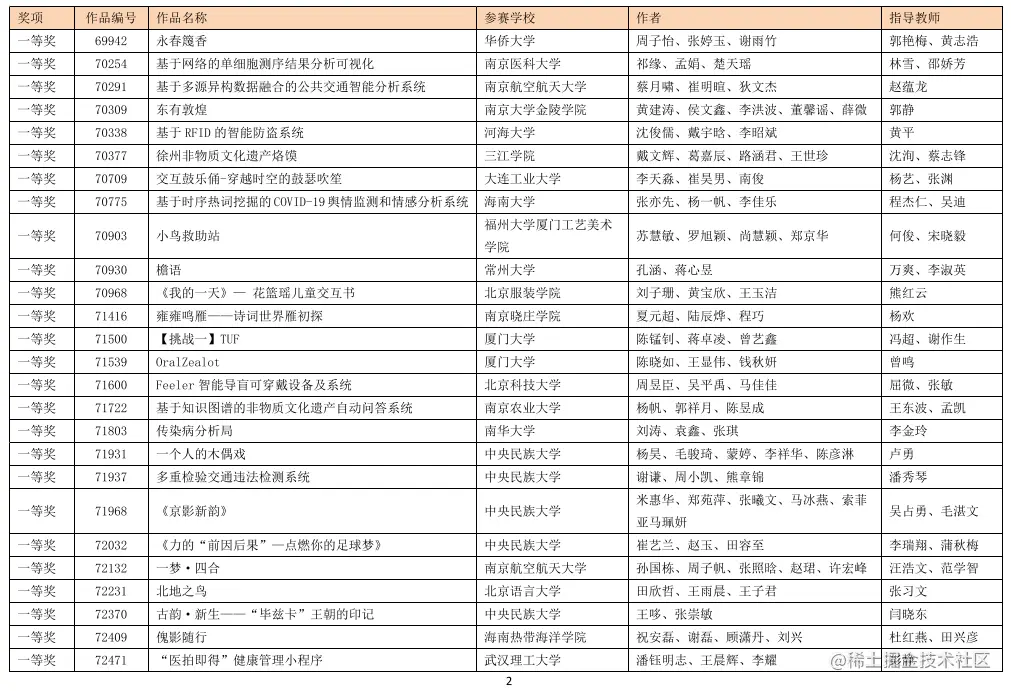Next `PDF` Extract the table in , And save to `Excel` in .

First import the required modules ：

``````import pdfplumber
import pandas as pd
Copy code ``````

Read `PDF` file

``````read_path = '2020 List of winners of entries in China University Student Computer Design Competition .pdf'
Copy code ``````

`pages` Attribute contains `PDF` Information per page in , Cycle content per page , Use `extract_table()` Method to extract the table data in each page , And turn the data into `DataFrame`, Finally, merge the data on each page .

``````result_df = pd.DataFrame()
for page in pdf_2020.pages:
table = page.extract_table()
df_detail = pd.DataFrame(table[1:], columns=table)
#  Merge data sets per page
result_df = pd.concat([df_detail, result_df], ignore_index=True)
Copy code ``````

here DataFrame The data are as follows ：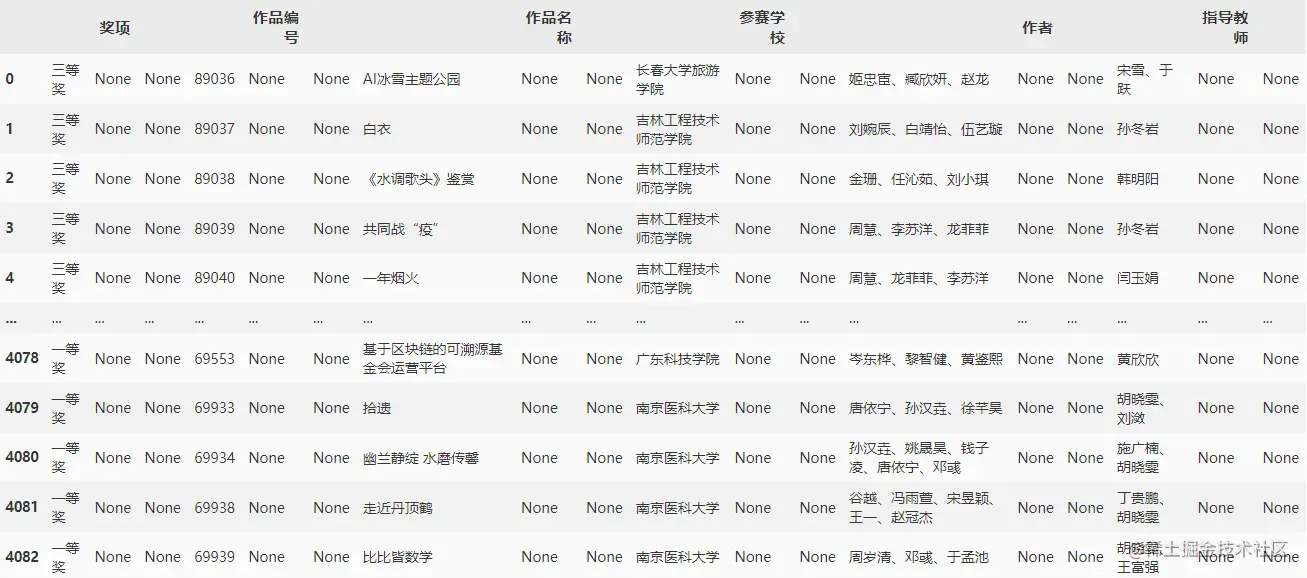You can see through `extract_table()` The extracted data has many columns with missing values , We need to be right about DataFrame Further processing , Delete all columns with missing values .

``````result_df.dropna(axis=1, how='all', inplace=True)
Copy code ``````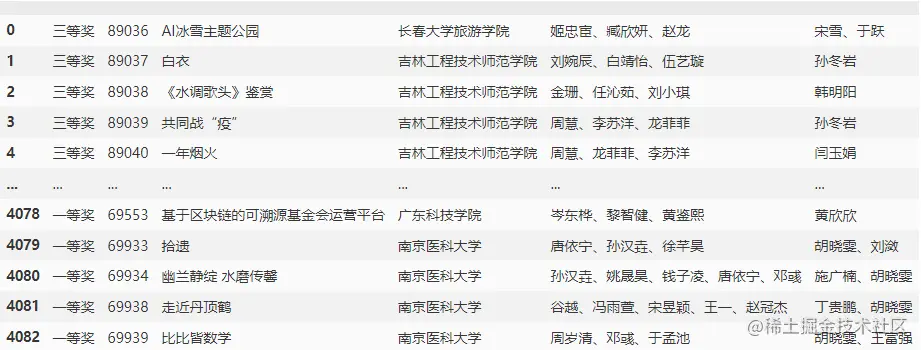After deleting the missing value , The column names are also deleted , You also need to specify the corresponding column name .

``````result_df.columns = [' prize ', ' Work number ', ' The title of the work ', ' Participating schools ', ' author ', ' The instructor ']
Copy code ``````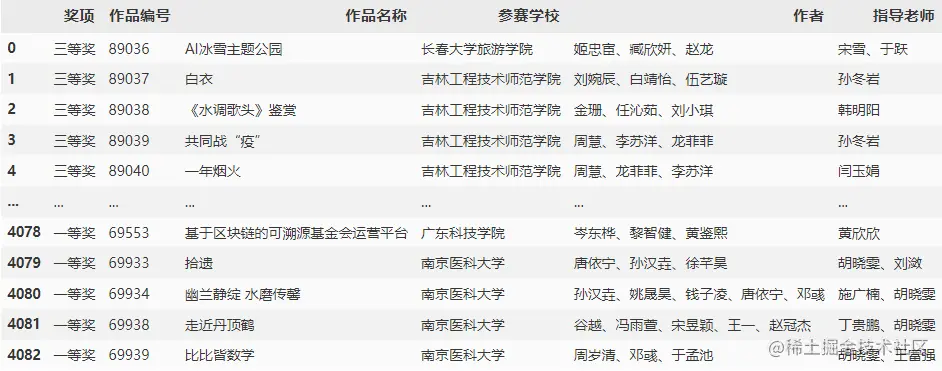Up to now, we have successfully extracted the table information completely ！

### Complete code

``````import pdfplumber
import pandas as pd

result_df = pd.DataFrame()
for page in pdf_2020.pages:
table = page.extract_table()
print(table)
df_detail = pd.DataFrame(table[1:], columns=table)
result_df = pd.concat([df_detail, result_df], ignore_index=True)
result_df.dropna(axis=1, how='all', inplace=True)
result_df.columns = [' prize ', ' Work number ', ' The title of the work ', ' Participating schools ', ' author ', ' The instructor ']
result_df.to_excel(excel_writer=save_path, index=False, encoding='utf-8')

read_path = r'2020 List of winners of entries in China University Student Computer Design Competition .pdf'
save_path = r'2020 List of winners of entries in China University Student Computer Design Competition .xlsx'
Copy code ``````

That's what I want to share today , Wechat search  Python New horizons , Take you to learn more useful knowledge every day . There are nearly 1000 sets of resume templates , Hundreds of e-books are waiting for you to pick them up ！ And then there is Python Xiaobai communication group , If you are interested, you can contact me through the above way ！